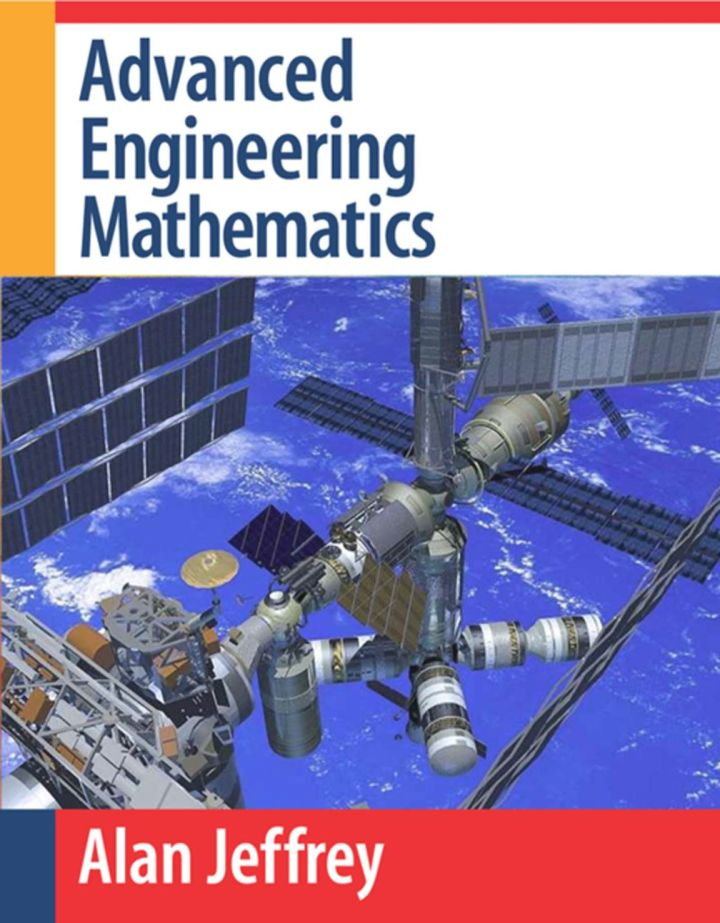# Advanced Engineering Mathematics

## Advanced Engineering Mathematics

By Jeffrey, Alan

### Rent or Buy eTextbook

Expires on Apr 19th, 2022
\$123

Publisher List Price: \$144.99
Savings: \$21.99

Advanced Engineering Mathematics provides comprehensive and contemporary coverage of key mathematical ideas, techniques, and their widespread applications, for students majoring in engineering, computer science, mathematics and physics. Using a wide range of examples throughout the book, Jeffrey illustrates how to construct simple mathematical models, how to apply mathematical reasoning to select a particular solution from a range of possible alternatives, and how to determine which solution has physical significance. Jeffrey includes material that is not found in works of a similar nature, such as the use of the matrix exponential when solving systems of ordinary differential equations. The text provides many detailed, worked examples following the introduction of each new idea, and large problem sets provide both routine practice, and, in many cases, greater challenge and insight for students. Most chapters end with a set of computer projects that require the use of any CAS (such as Maple or Mathematica) that reinforce ideas and provide insight into more advanced problems. A Student Solutions Manual is also available.

* Comprehensive coverage of frequently used integrals, functions and fundamental mathematical results
* Contents selected and organized to suit the needs of students, scientists, and engineers
* Contains tables of Laplace and Fourier transform pairs
* New section on numerical approximation
* New section on the z-transform
* Easy reference system

Subject: Mathematics & Statistics -> Mathematics -> Mathematics GeneralPublisher: Elsevier S & T 06/2001
Imprint: Academic Press
Language: English
Length: 1184 pages

ISBN 10: 012382592X
ISBN 13: 9780123825926
Print ISBN: 9780123825926

Live Chats